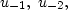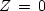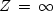Next: MINIMUM-PHASE FILTERS Up: INSTABILITY Previous: Boundedness

## Causality and the unit circle

The most straightforward way to say that a filter is causal is to say that its time-domain coefficients vanish before zero lag, that is, ut = 0 for t < 0. Another way to say this is U(Z) is finite for Z=0. At Z=0, the Z-transform would be infinite if the coefficientsetc., were not zero.

For a causal function, each term in U(Z) will be smaller if Z is taken to be inside the circle |Z| < 1 rather than on the rim |Z|=1. Thus, convergence at Z=0 and on the circle |Z|=1 implies convergence everywhere inside the unit circle. So boundedness combined with causality means convergence in the unit circle.

Convergence atbut not on the circlewould refer to a causal function with infinite energy, a case of no practical interest. What function converges on the circle, at, but not at? What function converges at all three places,,, and?Next: MINIMUM-PHASE FILTERS Up: INSTABILITY Previous: Boundedness
Stanford Exploration Project
10/21/1998